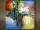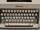Election mathematics

In elections, 12 political parties received this shares of voters:

party A 56.2 %
party B 8.5 %
party C 8.2 %
party D 6.2 %
party E 6.1 %
party F 5.5 %
party G 3.2 %
party H 2.1 %
party I 2 %
party J 1 %
party K 1 %

Calculate what the shares acquired in the parliament, if a quorum is 5%.

Result

A =  62 %
B =  9.4 %
C =  9 %
D =  6.8 %
E =  6.7 %
F =  6.1 %
G =  0 %
H =  0 %
I =  0 %
J =  0 %
K =  0 %

Solution:Leave us a comment of example and its solution (i.e. if it is still somewhat unclear...):Be the first to comment!To solve this example are needed these knowledge from mathematics:

Need help calculate sum, simplify or multiply fractions? Try our fraction calculator. Our percentage calculator will help you quickly calculate various typical tasks with percentages.

Next similar examples:

1. PipeSteel pipe has a length 2.5 meters. About how many decimetres is 1/3 less than 4/8 of this steel pipe?
2. DonutsFind how many donuts each student will receive if you share 126 donuts in a ratio of 1:5:8
3. IronIron ore contains 57% iron. How much ore is needed to produce 20 tons of iron?
4. ClassIn a class are 32 pupils. Of these are 8 boys. What percentage of girls are in the class?
5. Image scaleThe actual image dimensions are 60 cm x 80cm and has a reduced size 3 cm x 4 cm. At what scale the image was reduced?
6. TVsProduction of television sets increased from 3,500 units to 4,200 units. Calculate the percentage of production increase.
7. Percents - easyHow many percent is 432 out of 434?
8. New refrigeratorNew refrigerator sells for 1024 USD, Monday will be 25% discount. How much USD will save, and what will be the price?
9. Base, percents, valueBase is 344084 which is 100 %. How many percent is 384177?
10. GlovesI have a box with two hundred pieces of gloves in total, split into ten parcels of twenty pieces, and I sell three parcels. What percent of the total amount I sold?
11. ClassIn 7.C clss are 10 girls and 20 boys. Yesterday was missing 20% of girls and 50% boys. What percentage of students missing?
12. Seeds 2How many seeds germinated from 1000 pcs, when 23% no emergence?
13. Apples 2James has 13 apples. He has 30 percent more apples than Sam. How many apples has Sam?
14. PercentsHow many percents is 900 greater than the number 750?
15. NumberWhat number is 20 % smaller than the number 198?
16. Land areaA land area of Asia and Africa are in a 3: 2 ratio, the European and African are is 1:3. What are the proportions of Asia, Africa, and Europe?
17. Typing courseBefore taking a typing course, Terrence could type 39 words per minute. By the end of the course, he was able to type 68 words per minute. Find the percent increase.